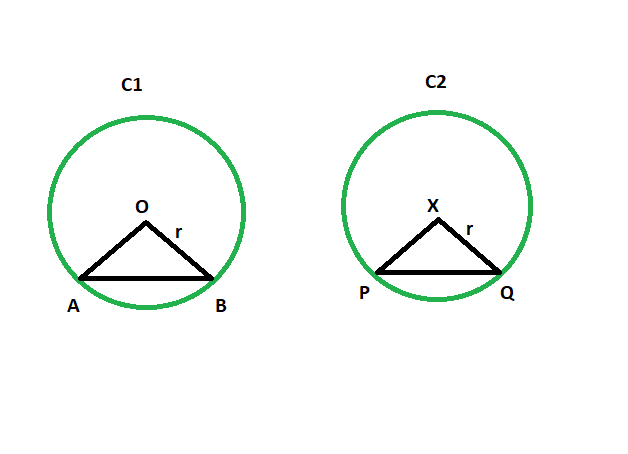Open in App
Not now

# Angle subtended by the chord to center of the circle when the angle subtended by the another equal chord of a congruent circle is given

• Last Updated : 30 May, 2022

Given are two congruent circles with two equal chords. The angle subtended to the center from the chord of one of the circles is given. The task is to find the angle subtended by the chord to the center of another circle.
Examples:

```Input: z = 48
Output: 48 degrees

Input: z = 93
Output: 93 degrees```Approach

• In triangle AOB and PXQ

```AO = PX(radii of congruent circles)
BO = QX(radii of congruent circles)
AB = PQ(equal chords)```
•
• So, triangle AOB is congruent with triangle PXQ

• So, angle AOB = angle PXQ

Equal chords of congruent circles subtend equal angles at their centers.

Below is the implementation of the above approach:

## C++

 `// C++ program to find the angle subtended by the chord` `// to the centre of the circle when the angle subtended` `// by another equal chord of a congruent circle is given`   `#include ` `using` `namespace` `std;`   `void` `anglequichord(``int` `z)` `{` `    ``cout << ``"The angle is "` `<< z` `         ``<< ``" degrees"` `<< endl;` `}`   `// Driver code` `int` `main()` `{` `    ``int` `z = 48;` `    ``anglequichord(z);` `    ``return` `0;` `}`

## Java

 `// Java program to find the angle subtended by the chord` `// to the centre of the circle when the angle subtended` `// by another equal chord of a congruent circle is given`   `import` `java.io.*;`   `class` `GFG ` `{` `    `  `static` `void` `anglequichord(``int` `z)` `{ ` `    ``System.out.println (``"The angle is "` `+ z + ``" degrees"``);` `}`   `// Driver code` `public` `static` `void` `main (String[] args) ` `{`   `    ``int` `z = ``48``;` `    ``anglequichord(z);`   `}` `}`

## Python 3

 `# Python 3 program to find the angle subtended by the chord` `# to the centre of the circle when the angle subtended` `# by another equal chord of a congruent circle is given`     `def` `anglequichord(z):` `    ``print` `(``"The angle is "` `, z` `        ``, ``" degrees"``)`   `# Driver code` `if` `__name__ ``=``=` `"__main__"``:` `    ``z ``=` `48` `    ``anglequichord(z)`   `# This code is contributed by ChitraNayal`

## C#

 `// C# program to find the angle subtended by the chord ` `// to the centre of the circle when the angle subtended ` `// by another equal chord of a congruent circle is given ` `using` `System;`   `class` `GFG ` `{ ` `    `  `    ``static` `void` `anglequichord(``int` `z) ` `    ``{ ` `        ``Console.WriteLine(``"The angle is "` `+ z + ``" degrees"``); ` `    ``} ` `    `  `    ``// Driver code ` `    ``public` `static` `void` `Main () ` `    ``{ ` `    `  `        ``int` `z = 48; ` `        ``anglequichord(z); ` `    ``} ` `} `   `// This code is contributed by AnkitRai01`

## Javascript

 ``

Output:

`The angle is 48 degrees`

Time Complexity: O(1)

Auxiliary Space: O(1)

My Personal Notes arrow_drop_up
Related Articles